# Data-driven distributionally robust optimization (Mohajerin Esfahani and Kuhn, 2018)¶

This notebook illustrates parameterization in MOSEK's Fusion API for python, by implementing the numerical experiments presented in a paper titled "Data-driven distributionally robust optimization using the Wasserstein metric: performance guarantees and tractable reformulations" (Mohajerin Esfahani and Kuhn hereafter), authored by Peyman Mohajerin Esfahani and Daniel Kuhn.

# Data-driven stochastic programming (Paper summary)¶

Consider a loss function $h(x,\xi)$ which depends on the decision variables $x$ and uncertain parameters $\xi$. The goal is to minimize the expected value of $h(x,\xi)$ for all $x\in \mathbb{X}$, where $\mathbb{X} \subset \mathbb{R}^n$. The expected value is calculated over $\mathbb{P}(\xi)$, which is the probability distribution of the random vector $\xi \in \mathbb{R}^m$. This distribution is supported on the uncertainty set $\Xi$. The problem statement is written as in $\ref{eq:main_prob}$.

$$\begin{equation} J^\star := \underset{x\in \mathbb{X}}{\inf} \bigg\{ \mathbb{E}^{\mathbb{P}}\big[ h(x,\xi) \big] = \int_{\Xi} h(x,\xi)\mathbb{P}(d\xi)\bigg\} \label{eq:main_prob} \tag{1} \end{equation}$$

In practice, however, the probability distribution is often unknown. Instead, one usually has access to a set $\widehat{\Xi}_N := \{\widehat{\xi}_i \}_{i\leq N}$, that contains $N$ historic realizations of the $\xi$ vector. A data-driven solution $\widehat{x}_N$ can be constructed by using some approximation of the true probability distribution. A natural choice for such an approximation is based on $\widehat{\Xi}_N$, and it is given by the discrete empirical probability distribution, as shown in $\ref{eq:empirical_dist}$.

$$\begin{equation} \mathbb{\widehat{P}}_N := \frac{1}{N}\sum^{N}_{i = 1} \delta_{\widehat{\xi}_i} \label{eq:empirical_dist} \tag{2} \end{equation}$$

The sample-average-approximation, which is obtained by replacing $\mathbb{P}$ with $\mathbb{\widehat{P}}_N$ in $\ref{eq:main_prob}$, is usually easier to solve but shows poor out-of-sample performance. Out-of-sample performance of a data-driven solution $\widehat{x}_N$ is the expected loss for that decision under the true probability distribution. To obtain better out-of-sample performance, Mohajerin Esfahani and Kuhn solve the robust optimization problem stated in $\ref{eq:dist_rob}$.

$$\begin{equation} \widehat{J}_N := \underset{x\in \mathbb{X}}{\inf} \underset{\mathbb{Q}\in \mathcal{\widehat{P}}_N}\sup \mathbb{E}^\mathbb{Q}\big[ h(x, \xi)\big] \label{eq:dist_rob} \tag{3} \end{equation}$$

The distributionally robust problem stated in $\ref{eq:dist_rob}$ is conceptually similar to $\ref{eq:main_prob}$, but it compensates for the unknown $\mathbb{P}$ by maximizing the expected value over all probability distributions $\mathbb{Q}$ inside an ambiguity set $\widehat{\mathcal{P}}_N$. Maximization is done in order to obtain the worst-case estimate.

If $\widehat{x}_N$ is an optimal decision vector for the distributionally robust program, then the authors associate performance guarantees to $\widehat{x}_N$ of the type shown in $\ref{eq:perf_guarantee}$.

$$\begin{equation} \mathbb{P}^N \bigg\{ \widehat{\Xi}_N : \mathbb{E}^\mathbb{P}\big[ h(\widehat{x}_N, \xi) \big] \leq \widehat{J}_N \bigg \} \geq 1 - \beta \label{eq:perf_guarantee} \tag{4} \end{equation}$$

Here, $\mathbb{P}^N$ is a probability distribution that governs the realization of the set $\widehat{\Xi}_N$. The expression in $\ref{eq:perf_guarantee}$ states that $\widehat{J}_N$ provides an upper bound to the out-of-sample performance of $\widehat{x}_N$ with at least $1-\beta$ probability. Thus, $\widehat{J}_N$ is a certificate of out-of-sample performance and the probability on the left side is the reliability of this certificate.

### Choice of the ambiguity set $\widehat{\mathcal{P}}_N$¶

The ambiguity set resides within a space of probability distributions and is chosen to be a ball of radius $\epsilon$ centered at $\widehat{\mathbb{P}}_N$. The metric of choice is the Wasserstein metric. Mathematically, the ambiguity set is defined as shown in $\ref{eq:wass_ball}$.

$$\begin{equation} \mathbb{B}_\epsilon(\mathbb{\widehat{P}}_N) := \big\{ \mathbb{Q} \in \mathcal{M}(\Xi) : d_W (\mathbb{\widehat{P}}_N, \mathbb{Q}) \leq \epsilon \big\} \label{eq:wass_ball} \tag{5} \end{equation}$$

As proved in section 3 of the paper, solving $\ref{eq:dist_rob}$ with $\mathcal{\widehat{P}}_N$ set to $\mathbb{B}_\epsilon(\mathbb{\widehat{P}}_N)$ will provide finite sample performance guarantees of the type seen in $\ref{eq:perf_guarantee}$. Furthermore, given certain assumptions (see paper), as $N\to\infty$, the certificate $\widehat{J}_N$ approaches $J^\star$ (optimal value of $\ref{eq:main_prob}$) and the data-driven decision $\widehat{x}_N$ approaches the true optimal decision $x^\star$.

### Convex reduction of the worst-case expectation problem¶

The infinite dimensional maximization problem within $\ref{eq:dist_rob}$ can be reduced to a computationally tractable finite-dimensional convex program, given the following requirements are met:

1.) The uncertainty set $\Xi$ is convex and closed.

2.) The loss function is a point-wise maximum of more elementary functions, i.e. $l(\xi) := \underset{k\leq K}{\max} l_k(\xi)$, where $-l_k(\xi)$ are proper, convex, and lower semi-continuous for all $k\leq K$. Note the suppression of the loss function's dependence on $x$.

For problems that satisfy these requirements, the convex reduction is given in $\ref{eq:convex_red}$. \begin{align} & \underset{\lambda, s_i, z_{ik}, \nu_{ik}}{\inf} & & \lambda \epsilon + \frac{1}{N}\sum_{i=1}^{N}s_i & \nonumber \\ & \text{s.t.} & &[-l_k]^*(z_{ik} - \nu_{ik}) + \sigma_{\Xi}(\nu_{ik}) - \langle z_{ik},\widehat{\xi}_i\rangle \leq s_i & & \forall i\leq N , \forall k\leq K \label{eq:convex_red} \tag{6}\ \\ & & &\|z_{ik}\|_* \leq \lambda & & \forall i\leq N, \forall k\leq K \nonumber \\ \end{align}

where $[-l_k]^*$ denotes the conjugate of the negative $k^{\text{th}}$ component of the loss function, $\|z_{ik}\|_*$ is the dual norm corresponding to the norm used in defining the Wasserstein ball and $\sigma_\Xi$ is the support function for the uncertainty set.

# Mean risk Portfolio Optimization (Section 7.1 in paper)¶

Consider a market that forbids short-selling and has $m$ assets. Yearly returns of these assets are given by the random vector $\xi = [\xi_1, ..., \xi_m]^T$. The percentage weights (of the total capital) invested in each asset are given by the decision vector $x = [x_1, ..., x_m]^T$ where $x$ belongs to $\mathbb{X} = \{x \in \mathbb{R}_+^m : \sum^{m}_{i=1}x_i = 1\}$. We have to solve the problem stated in $\ref{eq:mean_risk_port}$.

$$\begin{equation} J^\star = \underset{x\in \mathbb{X}}{\inf} \big\{ \mathbb{E}^{\mathbb{P}}[-\langle x, \xi \rangle] + \rho \mathbb{P}\text{-CVaR}_\alpha \big(-\langle x, \xi\rangle\big)\big\} \label{eq:mean_risk_port} \tag{7} \end{equation}$$

which amounts to minimizing a weighted sum of the expected value and the conditional value-at-risk (see also: Fusion notebook on stochastic risk measures) of the portfolio loss, $- \langle x, \xi \rangle$. Using the definition of conditional value-at-risk (Optimization of conditional value-at-risk by R. T. Rockafellar and S. Uryasev), $\ref{eq:mean_risk_port}$ can be written as a maximum of $K=2$ affine loss functions ($\ref{eq:cvar_prob}$).

$$\begin{equation} J^\star = \underset{x \in \mathbb{X}, \tau \in \mathbb{R}}{\inf} \mathbb{E}^{\mathbb{P}}\big[\underset{k\leq K}{\max} a_k \langle x, \xi \rangle + b_k \tau \big] \label{eq:cvar_prob} \tag{8} \end{equation}$$$$\text{where}\,\, a =\big(-1, -1-\frac{\rho}{\alpha}\big)\, ,\,\, b =\big(\rho, \rho-\frac{\rho}{\alpha}\big)$$

If the uncertainty set is a polytope of the form $\Xi = \{ \xi \in \mathbb{R}^m : C\xi \leq d \}$, then the distributionally robust counterpart of the program in $\ref{eq:cvar_prob}$ will have a convex reduction as shown in $\ref{eq:convex_red_port}$.

\begin{align} & \underset{x,\tau,\lambda,s_i, \gamma_{ik}}{\inf} & & \lambda \epsilon + \frac{1}{N}\sum^{N}_{i=1}s_i & \nonumber \\ &\text{s.t.} & & b_k \tau + a_k \langle x, \widehat{\xi}_i \rangle + \langle \gamma_{ik}, d - C\widehat{\xi}_i\rangle \leq s_i & \forall i\leq N, \forall k\leq K \label{eq:convex_red_port} \tag{9}\\ & & &\| C^T \gamma_{ik} - a_k x\|_* \leq \lambda & \forall i\leq N, \forall k\leq K &\nonumber \\ & & & \gamma_{ik} \geq 0 & \forall i \leq N, \forall k \leq K & \nonumber \\ & & & x \in \mathbb{X} & \nonumber \\ \end{align}

### Parameterized Fusion model¶

Finally, we make a Fusion model for the program in $\ref{eq:convex_red_port}$. The following code cell defines DistributionallyRobustPortfolio, a wrapper class (around our fusion model) with some convenience methods. The key steps in the Fusion model implementation are (see portfolio_model method in class):

• M = Model('DistRobust_m{0}_N{1}'.format(m, N)): instantiate a Fusion Model object.
• dat = M.parameter('TrainData', [N, m]) and eps = M.parameter('WasRadius'): declare a Fusion parameter object named "TrainData" and a parameter for the Wasserstein Radius, called "WasRadius". "TrainData" represents the past N realizations of the m sized random vector, $\xi$.
• a_k = [-1, -51] and b_k = [10, -40]: lists that are defined as in $\ref{eq:cvar_prob}$. All experiments that follow have the same $a_k$ and $b_k$, therefore we use lists. However, with minor modifications these lists can be replaced with Fusion parameters.
• x = M.variable('Weights', m, Domain.greaterThan(0.0)): declare Fusion variables for all the optimization variables involved in $\ref{eq:convex_red_port}$, except $\gamma$, which is excluded as $\Xi \in \mathbb{R}^m$ (see the market setup below).
• M.objective('J_N(e)', ObjectiveSense.Minimize, certificate): declare the objective sense and the expression of the objective. Here certificate is an Expr object that evaluates to the objective in $\ref{eq:convex_red_port}$.
• self.M.constraint('C1',Expr.add([e1, e2, Expr.neg(Expr.repeat(s, 2, 1))]), Domain.lessThan(0.0)): defining the constraints. Here e1 and e2 are Expr objects that evaluate to the relevant terms in the first constraint in $\ref{eq:convex_red_port}$. Constraints "C2_pos" and "C2_neg" correspond to the second constraint and finally "C3" ensures that $x \in \mathbb{X}$.
• self.M.setSolverParam('optimizer','freeSimplex'): use the simplex optimizer. By default, MOSEK will use its interior point optmizer. However, we plan to re-solve a linear model many times over and it is therefore imperative to exploit the warm-start capabilities of the simplex optimizer.

Note: More details regarding the code can be found within comments.

In :
class DistributionallyRobustPortfolio:

def __init__(self, m, N):
self.m, self.N = m, N
self.M = self.portfolio_model(m, N)
self.x = self.M.getVariable('Weights')
self.t = self.M.getVariable('Tau')
self.dat = self.M.getParameter('TrainData')
self.sol_time = []

def portfolio_model(self, m, N):
'''
Parameterized Fusion model for program in 9.
'''
M = Model('DistRobust_m{0}_N{1}'.format(m, N))
##### PARAMETERS #####
dat = M.parameter('TrainData', [N, m])
a_k = [-1, -51]  # Alternative: Fusion parameters.
b_k = [10, -40]  # Alternative: Fusion parameters.
##### VARIABLES #####
x = M.variable('Weights', m, Domain.greaterThan(0.0))
s = M.variable('s_i', N)
l = M.variable('Lambda')
t = M.variable('Tau')
##### OBJECTIVE #####
# certificate = lamda*epsilon + sum(s)/N
Expr.mul(1/N, Expr.sum(s)))
M.objective('J_N(e)', ObjectiveSense.Minimize, certificate)
##### CONSTRAINTS #####
# b_k*t
e1 = Expr.transpose(Expr.repeat(Expr.mul(t, b_k), N, 1))
# a_k*<x,xi>
e2 = Expr.hstack([Expr.mul(a_k[i], Expr.mul(dat, x))
for i in range(2)])
# b_k*t + a_k*<x,xi> <= s
[e1, e2, Expr.neg(Expr.repeat(s, 2, 1))]), Domain.lessThan(0.0))
# a_k*x
e3 = Expr.hstack([Expr.mul(a_k[i], x) for i in range(2)])
e4 = Expr.repeat(Expr.repeat(l, m, 0), 2, 1)
# ||a_k*x||_infty <= lambda
M.constraint('C2_pos', Expr.sub(e3, e4), Domain.lessThan(0.0))
# x \in X
M.constraint('C3', Expr.sum(x), Domain.equalsTo(1.0))
# Use the simplex optimizer
M.setSolverParam('optimizer', 'freeSimplex')
return M

def sample_average(self, x, t, data):
'''
Calculate the sample average approximation for given x and tau.
'''
l = np.matmul(data, x)
return np.mean(np.maximum(-l + 10*t, -51*l - 40*t))

def iter_data(self, data_sets):
'''
Generator method for iterating through values for the
TrainData parameter.
'''
for data in data_sets:
yield self.simulate(data)

'''
Generator for iterating through values for the WasRadius
parameter.
'''
for epsilon in epsilon_range:
yield self.solve(epsilon)

def simulate(self, data):
'''
Tentative goal of this method is to set a value for the
data parameter, and solve the model for the same.

Define in child classes.
'''
pass

def solve(self, epsilon):
'''
Tentative goal of this method is to set a value for the
radius parameter, and solve the model for the same.

Define in child classes.
'''
pass


### Market setup¶

The simulation results presented in the paper are based on a market with $m = 10$ assets. Each asset's return is expressed as $\xi_i = \psi + \zeta_i$, where $\psi \sim \mathcal{N}(0, 0.02)$ is the systematic risk factor and $\zeta_i \sim \mathcal{N}(0.03\times i, 0.025\times i)$ is the idiosyncratic risk factor. By construction, assets with higher indices have higher risk as well as higher returns. The uncertainty set is $\Xi \in \mathbb{R}^m$, thus $C$ and $d$ are set to zero. $1$-norm is used to define the Wasserstein ball, therefore the dual norm will be the $\infty$-norm. Lastly, $\alpha = 20\%$ and $\rho = 10$ are used within the loss function.

In :
# Function to generate N samples of the m-shaped random vector xi.
def normal_returns(m, N):
R = np.vstack([np.random.normal(
i*0.03, np.sqrt((0.02**2+(i*0.025)**2)), N) for i in range(1, m+1)])
return (R.transpose())


# Simulations and results¶

The concluding section of this notebook reproduces the results provided in sections 7.2 of Mohajerin Esfahani and Kuhn. We do not pursue details of the design of these simulations, instead we focus primarily on implementing them with the models constructed above. The reader will find details/hints about the code within the in-code comments.

## Impact of the Wasserstein radius¶

In :
import numpy as np
from mosek.fusion import Model, Expr, Domain, ObjectiveSense
import matplotlib.pyplot as plt
from scipy.special import erfinv
from sklearn.model_selection import train_test_split
from sklearn.utils import resample

In :
# Inherits from the portfolio class defined above.
class SimSet1(DistributionallyRobustPortfolio):
# Market setup
m = 10
mu = np.arange(1, 11)*0.03
var = 0.02 + (np.arange(1, 11)*0.025)

# Constants for CVaR calculation.
beta, rho = 0.8, 10
c2_beta = 1/(np.sqrt(2*np.pi)*(np.exp(erfinv(2*beta - 1))**2)*(1-beta))

def __init__(self, N, eps_range):
self.eps_range = eps_range
# Fusion model instance
super().__init__(SimSet1.m, N)

def simulate(self, data):
'''
This method is called within the generator method called iter_data, which
was defined in the parent class.

Returns
wts: optimal asset weights for each radius
perf: analytic out-of-sample performance for each radius
'''
# Set TrainData parameter
self.dat.setValue(data)
# Iterate through range of Wasserstein radii (see solve method below)
wts, perf, rel = zip(*[(_w, _p, _r)
for _w, _p, _r in self.iter_radius(self.eps_range)])
return wts, perf, rel

def solve(self, epsilon):
'''
This method is used within the generator method called iter_radius, which
was defined in the parent class.

Returns:
x_sol: asset weights
out_perf: analytica out-of-sample performance
rel: reliability for the certificate
'''
self.eps.setValue(epsilon)
# Solve the Fusion model
self.M.solve()
self.sol_time.append(self.M.getSolverDoubleInfo('optimizerTime'))
# Portfolio weights
x_sol = self.x.level()
# Analytical out-of-sample performance
out_perf = self.analytic_out_perf(x_sol)
return x_sol, out_perf, (out_perf <= self.M.primalObjValue())

def analytic_out_perf(self, x_sol):
'''
Method to calculate the analytical value for the out-of-sample performance.
[see Rockafellar and Uryasev]
'''
mean_loss = -np.dot(x_sol, SimSet1.mu)
sd_loss = np.sqrt(np.dot(x_sol**2, SimSet1.var))
cVaR = mean_loss + (sd_loss*SimSet1.c2_beta)
return mean_loss + (SimSet1.rho*cVaR)

def run_sim(self, data_sets):
'''
Method to iterate over several datasets and record the results.
'''
wts, perf, rel = zip(*self.iter_data(data_sets))
self.weights = np.mean(wts, axis=0)
self.perf_mu = np.mean(perf, axis=0)
self.perf_20 = np.quantile(perf, 0.2, axis=0)
self.perf_80 = np.quantile(perf, 0.8, axis=0)
self.reliability = np.mean(rel, axis=0)

In :
# Cardinality
N = 300
# Number of simulations
num_sim = 200

# Range of Wasserstein radii to consider
epsilon_range = np.append(np.concatenate(
[np.arange(1, 10)*10.0**(i) for i in range(-4, 0)]), 1.0)

# Making 200 independent datasets
sim_data = [normal_returns(10, N) for i in range(num_sim)]

# Instantiate SimSet1 class
sim1 = SimSet1(N, epsilon_range)

# 200 simulations...
sim1.run_sim(sim_data)

print("Time taken in initial solve of model with N={0}: {1:.4f} s".format(
N, sim1.sol_time))
print("Mean time to solve a model with N={0}: {1:.4f} s".format(
N, np.mean(sim1.sol_time)))
print("Total time to solve 200 models with N={0}: {1:.4f} s".format(
N, np.sum(sim1.sol_time)))

Time taken in initial solve of model with N=300: 0.0064 s
Mean time to solve a model with N=300: 0.0018 s
Total time to solve 200 models with N=300: 13.0416 s

In :
fig, ax = plt.subplots(ncols=2, figsize=(10, 4), dpi=150, gridspec_kw={'wspace': 0.3})
fig.suptitle('N = {}'.format(N))

# Portfolio weight vs Wasserstein radius
for i in range(1, 11):
ax.fill_between(epsilon_range, np.sum(sim1.weights[:, :i-1], axis=1),
np.sum(sim1.weights[:, :i], axis=1),color=plt.cm.RdYlBu(1 - i/11))
ax.plot(epsilon_range, np.sum(sim1.weights[:, :i], axis=1),
color='black', linewidth=1.0)
ax.set_xscale('log')
ax.set_xlabel('$\epsilon$')
ax.set_xlim(10**-3, 1)
ax.set_ylim(0, 1)
ax.set_ylabel('Mean portfolio weights')

# Evolution of certificate and reliability
ax.plot(epsilon_range, sim1.perf_mu, color='blue')
ax.fill_between(epsilon_range, sim1.perf_20, sim1.perf_80, color='blue', alpha=0.4)
ax.set_xscale('log')
ax.set_xlabel('$\epsilon$')
ax.set_xlim(10**-4, 1.0)
ax.set_ylabel('Out-of-sample performance', color='blue')
ax.set_yticks(np.arange(-1.4, -0.8, 0.1))
ax.grid(which='major',alpha=0.4)
ax.grid(which='minor',alpha=0.3)

ax2 = ax.twinx()

ax2.set_ylabel('Reliability', color='red')
ax2.plot(epsilon_range, sim1.reliability, color='red')
ax2.set_yticks(np.arange(0, 1.2, 0.2))
ax2.set_ylim(0, 1)

plt.savefig('sim1_N{}.jpg'.format(N), dpi = 150, bbox_inches='tight')
plt.show()
plt.show()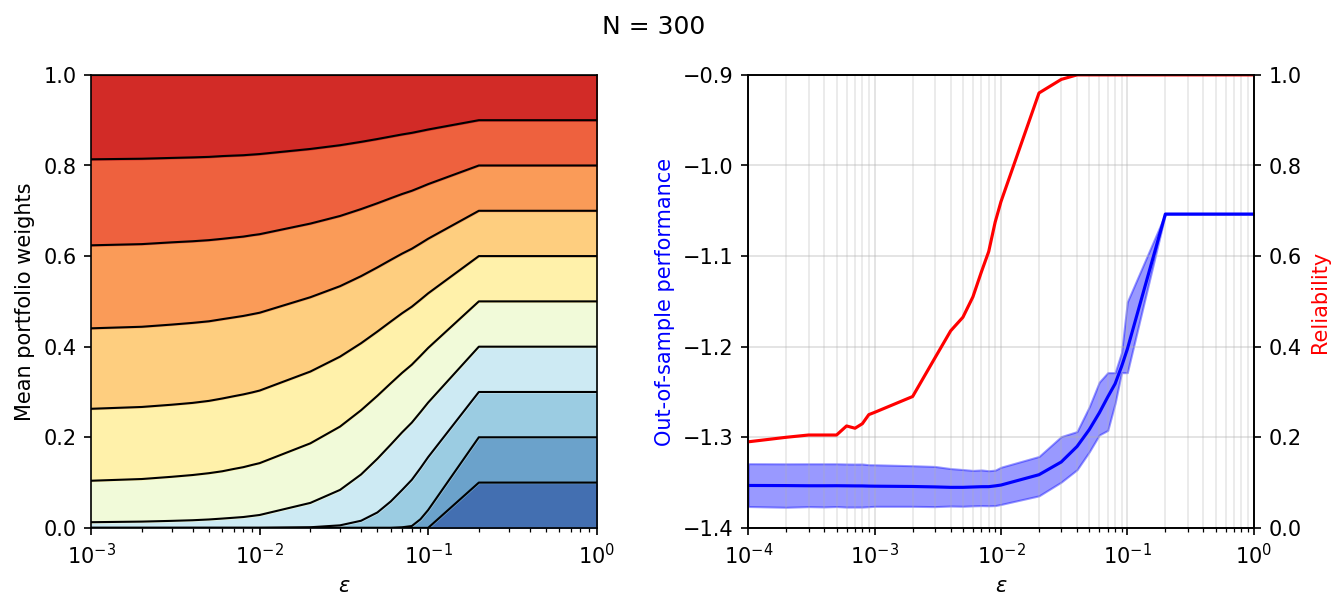## Portfolios driven by out-of-sample performance¶

### Holdout method¶

In :
class SimSet2_Holdout(DistributionallyRobustPortfolio):
# Market setup
m = 10

# Radius range (see page 156 in Mohajerin Esfahani and Kuhn)
eps_range = np.concatenate([np.arange(1, 10)*10.0**(i)
for i in range(-3, 0)])

# Validation data set of 2*10**5 samples (page 158 in paper)
valids = normal_returns(10, 2*10**5)

def __init__(self, N, k=5):
# 1/k sized split for the test data.
self.k = k
# Fusion model for (train) data size N*(k-1)/k (see holdout method)
super().__init__(SimSet2_Holdout.m, np.rint(N*(k-1)/k).astype(np.int32))

def validate(self, data_sets):
'''
Method to iterate over a list of independent datasets via the iter_data
generator method so as to apply the holdout technique to each dataset
and then save the results.
'''
self.rel = np.mean(np.array(self.perf) <= np.array(self.cert), axis=0)

def simulate(self, data):
'''
Method called within the iter_data generator.

Returns
out_perf: out-of-sample performance calculated with validation data
cert: performance certificate (optimal objective for M)
eps_holdout: radius selected from holdout method
'''
# Split data into test and train
train, self.test = train_test_split(data, test_size=1/self.k)
# Set the TrainData parameter to train data
self.dat.setValue(train)
# Iterate through a range of Wasserstein radii
out_perf_test, x, t, J_N = zip(
# Index of eps_holdout in the eps_range.
min_arg = np.argmin(out_perf_test)
# Out-of-sample performance for x_N(eps_holdout)
out_perf = self.sample_average(
x[min_arg], t[min_arg], SimSet2_Holdout.valids)
# J_N(eps_holdout)
cert = J_N[min_arg]
return out_perf, cert, SimSet2_Holdout.eps_range[min_arg]

def solve(self, epsilon):
'''
Method called within the iter_radius generator.

Returns
out_perf: SA-approx of out-of-sample performance using test data
x: Portfolio weights
t: Tau
self.M.primalObjValue(): performance certificate
'''
self.eps.setValue(epsilon)
# Solve the Fusion model
self.M.solve()
self.sol_time.append(self.M.getSolverDoubleInfo('optimizerTime'))
# Weights and Tau optimal values
x, t = self.x.level(), self.t.level()
# SAA of out-of-sample performance based on test data
out_perf = self.sample_average(x, t, self.test)
return out_perf, x, t, self.M.primalObjValue()

In :
# Range of cardinality values to consider
N_range = np.append(np.concatenate([np.arange(1,10)*10**(i) for i in range(1,3)]),1000)

holdout_results = []
for N in N_range:
# List of 200 independent datasets of cardinality N
n_data = [normal_returns(10, N) for i in range(200)]
# Instance of SimSet2_Holdout class with cardinality N
hld = SimSet2_Holdout(N)
# Running the holdout method over each dataset
hld.validate(n_data)
# Save the object
holdout_results.append(hld)

In :
def N_plot(results, title, save_name, y_l = [[-1.5, 0.5], [-2.5, 10], [0, 1]]):
N_range = np.append(np.concatenate(
[np.arange(1, 10)*10**(i) for i in range(1, 3)]), 1000)

fig, ax = plt.subplots(nrows=1, ncols=3, figsize=(13, 3), dpi=150)
fig.suptitle(title)
# Out-of-sample performance
ax.fill_between(N_range, [np.quantile(r.perf, 0.2, axis=0) for r in results], [
np.quantile(r.perf, 0.8, axis=0) for r in results], alpha=0.4)
ax.plot(N_range, [np.mean(r.perf, axis=0) for r in results], marker='>')
# Certificate
ax.fill_between(N_range, [np.quantile(r.cert, 0.2, axis=0) for r in results], [
np.quantile(r.cert, 0.8, axis=0) for r in results], alpha=0.4)
ax.plot(N_range, [np.mean(r.cert, axis=0) for r in results], marker='>')
# Reliability
ax.plot(N_range, [r.rel for r in results], marker='>')

y_n = ["Out-of-sample performance", "Certificate", "Reliability"]
for i in range(3):
ax[i].set_ylabel(y_n[i])
ax[i].set_ylim(y_l[i])
ax[i].grid(which='major',alpha=0.4)
ax[i].grid(which='minor',alpha=0.3)
ax[i].set_xscale('log')
ax[i].set_xlabel('N')
ax[i].set_xlim([10, 1000])
ax.set_yticks([-1.5, -1, -0.5, 0, 0.5])
plt.savefig(save_name, dpi = 150, bbox_inches='tight')
plt.show()

In :
N_plot(holdout_results, 'Holdout Method', 'sim2_holdout.jpg')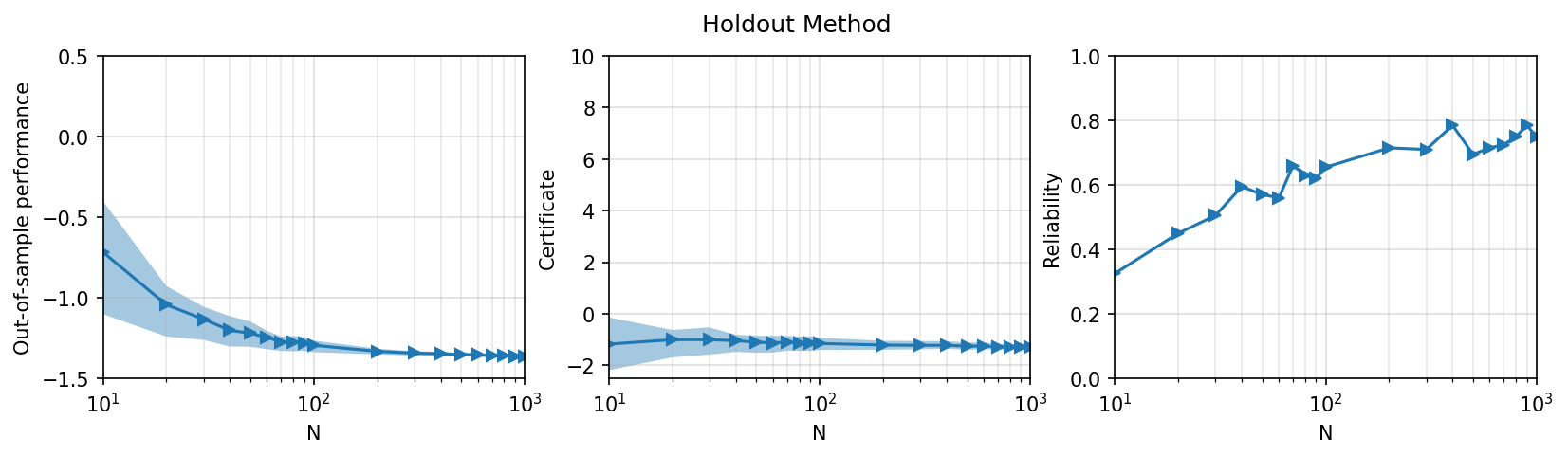### k-fold cross validation method¶

In :
# IMPORTANT: sub-class of the SimSet2_Holdout class!
class SimSet2_kFold(SimSet2_Holdout):

def __init__(self, N, k=5):
self.k = k
# Object for holdout method (k-holdouts)
super().__init__(N, k=k)
# Fusion model for N-size dataset (results)
self.M_N = self.portfolio_model(SimSet2_Holdout.m, N)
self.dat_N = self.M_N.getParameter('TrainData')
self.x_N = self.M_N.getVariable('Weights')
self.t_N = self.M_N.getVariable('Tau')

def simulate(self, data):
'''
Method called within the iter_data generator. This method overwrites
the one defined in the SimSet2_Holdout class.

Returns
out_perf: out-of-sample performance calculated with validation data
cert: performance certificate (optimal objective for M_N)
eps_kFold: radius selected from k-Fold method
'''
# Set TrainData paremeter for M_N to data
self.dat_N.setValue(data)
# Perform the holdout method k times and calculate eps_kFold
eps_kFold = np.mean([self._simulate(data) for i in range(self.k)])
# Set WasRadius to mean from k holdout runs
self.eps_N.setValue(eps_kFold)
# Solve the M_N model.
self.M_N.solve()
# Out-of-sample performance for x_N(eps_kFold)
out_perf = self.sample_average(
self.x_N.level(), self.t_N.level(), SimSet2_Holdout.valids)
# J_N(eps_kFold)
cert = self.M_N.primalObjValue()
return out_perf, cert, eps_kFold

def _simulate(self, data):
'''
Method to perform the holdout technique for a given dataset. This
is called k times within each call to the simulate method. Works
analogously to the simulate method of SimSet2_Holdout class.

Returns:
eps_holdout: WasRadius selected in one holdout run
'''
# Split data into test and train
train, self.test = train_test_split(data, test_size=1/self.k)
# Set TrainData parameter for the N*(k-1)/k model
self.dat.setValue(train)
# Solve N*(k-1)/k model iteratively for a range of radii
saa, x, t, J_N = zip(*self.iter_radius(SimSet2_Holdout.eps_range))
# Select Wasserstein radius that minimizes out-of-sample perf
min_arg = np.argmin(saa)
return SimSet2_Holdout.eps_range[min_arg]

In :
# Range of cardinality values to consider
N_range = np.append(np.concatenate([np.arange(1,10)*10**(i) for i in range(1,3)]),1000)

kFold_results = []

for N in N_range:
# List of 200 independent datdasets of cardinality N
n_data = [normal_returns(10,N) for i in range(200)]
# Instance of SimSet2_kFold class with cardinality N
kFld = SimSet2_kFold(N)
# Running the k-fold cross validation over each dataset
kFld.validate(n_data)
kFold_results.append(kFld)

In :
N_plot(kFold_results, 'k-fold cross validation method', 'sim2_kcross.jpg')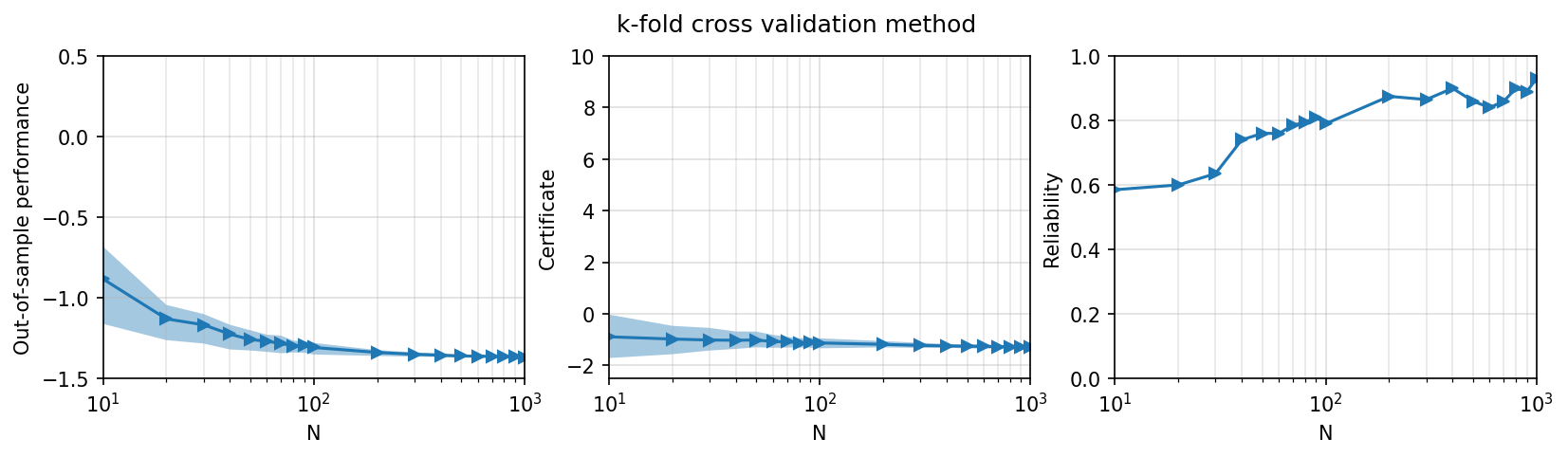## Portfolios driven by reliability¶

In :
class SimSet3(DistributionallyRobustPortfolio):
m = 10
eps_range = np.concatenate([np.arange(1, 10)*10.0**(i)
for i in range(-3, 0)])
valids = normal_returns(10, 2*10**5)

def __init__(self, beta, N, k=50):
# Number of resamples
self.k = k
# Reliability threshold
self.beta = beta
# Instantiate Fusion model
super().__init__(SimSet3.m, N)

def bootstrap(self, data_sets):
'''
Method to iterate over a list of independent datasets via the iter_data
generator method so as to apply the bootstrap technique to each dataset
and then save the results.
'''
self.rel = np.mean(np.array(self.perf) <= np.array(self.cert), axis=0)

def simulate(self, data):
'''
Method called within the iter_data generator.

Returns
out_perf: out-of-sample performance calculated with validation data
cert: performance certificate (optimal objective for M)
eps_btstrp: radius selected from holdout method
'''
# List to store reliability
rel = []
# Perform k resamples
for i in range(self.k):
# Split data into test and train
train, self.test = train_test_split(data, test_size=1/3)
# Resample train data up-to size N
train = resample(train, n_samples=self.N)
# Set TrainData parameter to train
self.dat.setValue(train)
# Iterate through a range of Wasserstein radii
# Sum reliability over all resamples (for each epsilon)
rel = np.sum(rel, axis=0)
# Smallest radius that has reliability over 1-beta
_id = next(i for i, r in enumerate(rel) if r >= self.k*(1-self.beta))
eps_btstrp = SimSet3.eps_range[_id]
# Set TrainData parameter to data
self.dat.setValue(data)
# Set WasRadius parameter to eps_btstrp
self.eps.setValue(eps_btstrp)
self.M.solve()
# Out-of-sample performance for x_N(eps_btstrp)
out_perf = self.sample_average(
self.x.level(), self.t.level(), SimSet3.valids)
cert = self.M.primalObjValue()
return out_perf, cert, eps_btstrp

def solve(self, epsilon):
'''
Method called within the iter_radius generator.

Returns
reliability: SAA of out-of-sample performance <= certificate(epsilon)
'''
# Set WasRadius parameter to epsilon and solve
self.eps.setValue(epsilon)
self.M.solve()
# Calculate out-of-sample performance SAA estimator using test
saa = self.sample_average(self.x.level(), self.t.level(), self.test)
# Boolean to state if the certificate is greater than SAA estimate
return saa <= self.M.primalObjValue()

In :
# Range of cardinality values to consider
N_range = np.append(np.concatenate(
[np.arange(1, 10)*10**(i) for i in range(1, 3)]), 1000)
# Reliability threshold: 1 - beta = 0.9
beta = 0.1

bootstrap_results = []
for N in N_range:
n_data = [normal_returns(10,N) for i in range(200)]
# Instance of SimSet3 class with cardinality N
btstrp = SimSet3(beta,N)
# Running the bootstrap method for each dataset
btstrp.bootstrap(n_data)
bootstrap_results.append(btstrp)

In :
N_plot(bootstrap_results, "1 - $\\beta$ = {}".format(1-beta), 'sim3_90.jpg',
y_l = [[-1.5, 1.5], [-2.5, 10], [0, 1]])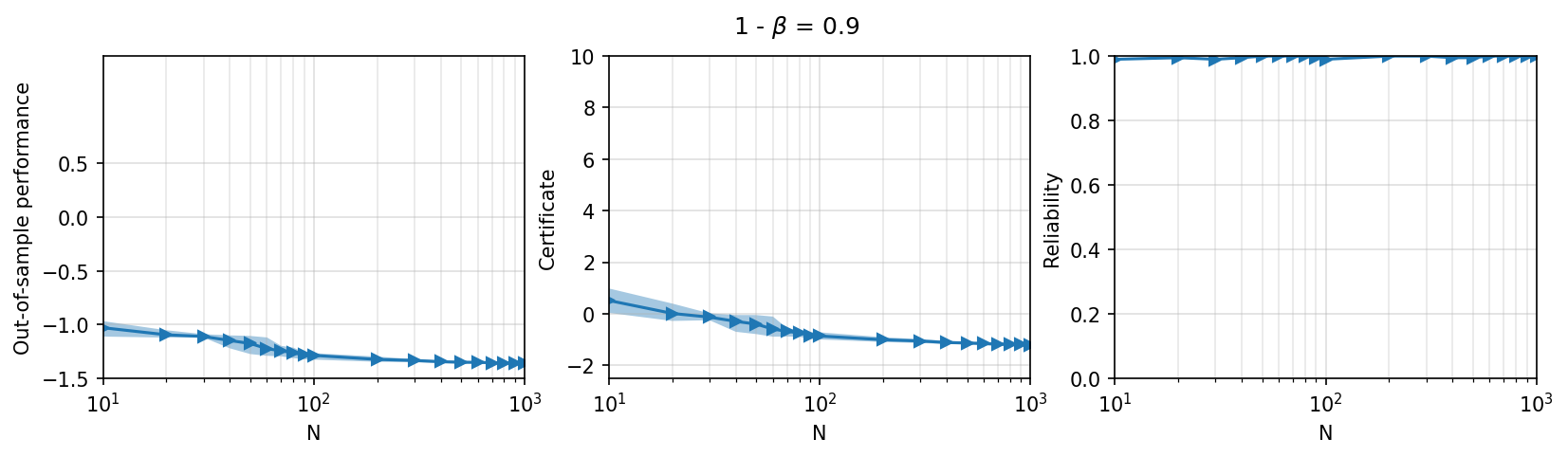## Impact of sample size on Wasserstein radius¶

In :
fig, ax = plt.subplots(figsize=(5, 4), dpi=150)
fig.suptitle('Impact of sample size on Wasserstein radius')

# Wasserstein radius vs sample size N
# HoldouN_rangeethod
ax.plot(N_range, [h.radius for h in holdout_results], color='blue',
linestyle='--', label='$\widehat{\epsilon}^{hm}_N$ Holdout')
# k-fold cross validation method
ax.plot(N_range, [k.radius for k in kFold_results], color='green',
marker='>', label='$\widehat{\epsilon}^{cv}_N$ k-fold')
# Bootstrap method (reliability driven portfolio)
ax.plot(N_range, [b.radii for b in bootstrap_results], color='tomato',
marker='<', label=r'$\widehat{\epsilon}^{\beta}_N$ $1 - \beta = 0.9$')

ax.set_xscale('log')
ax.set_yscale('log')
ax.set_xlabel('N')
ax.set_xlim(10, 1000)
ax.set_ylim(0.005, 0.5)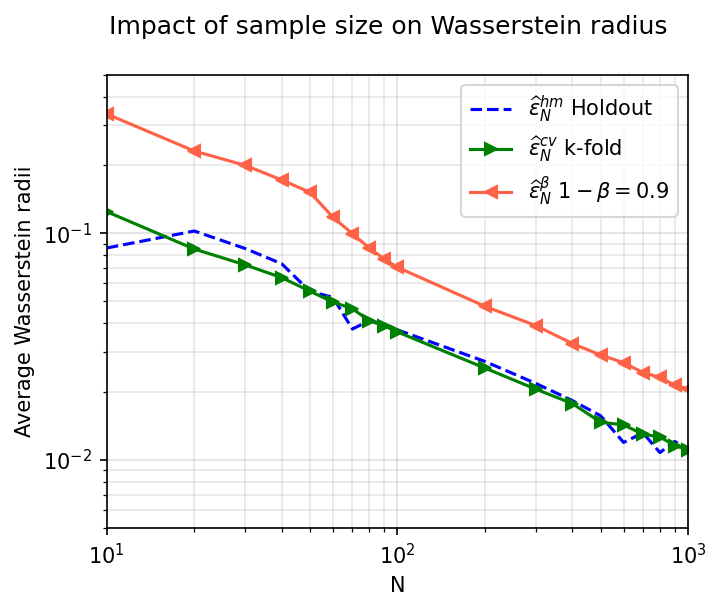This work is licensed under a Creative Commons Attribution 4.0 International License. The MOSEK logo and name are trademarks of Mosek ApS. The code is provided as-is. Compatibility with future release of MOSEK or the Fusion API are not guaranteed. For more information contact our support.